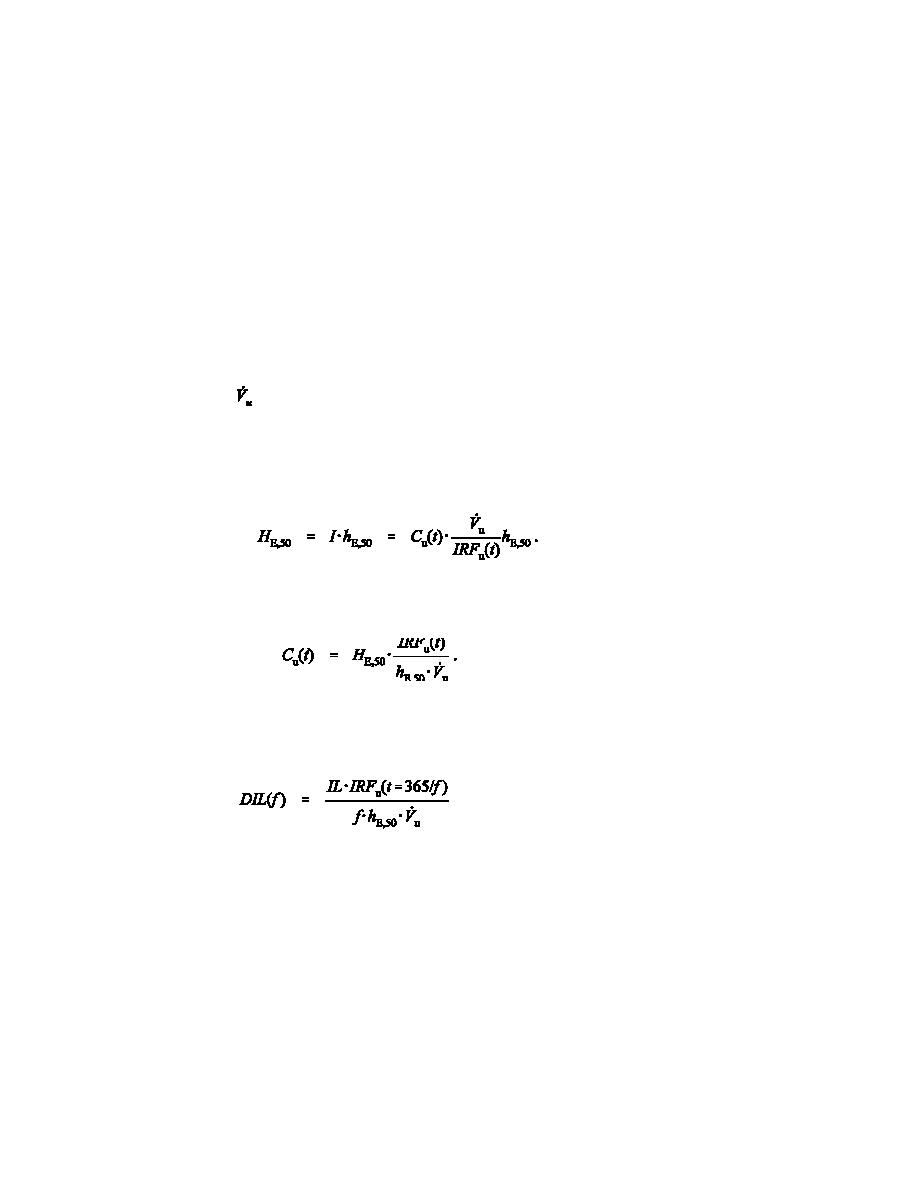Calculating the Derived Investigation Level for a Given Sample FrequencyClick here to make tpub.com your Home Page Page Title: Calculating the Derived Investigation Level for a Given Sample Frequency Back | Up | NextWeb www.tpub.comHome

Information Categories
Aerographer
Automotive
Aviation
Construction
Diving
Draftsman
Engineering
Electronics
Food and Cooking
Logistics
Math
Medical
Music
Nuclear Fundamentals
Photography
ReligionDOE-STD-1121-98
A second acceptable alternative is to compute a DIL as a function of sampling frequency. With a
sampling frequency of f samples per year (e.g., f = 12 per year for monthly samples), the goal of being
able to detect 100 mrem of HE,50 from all intakes in a year means that each analysis must be capable of
detecting 100 mrem ÷ f. Thus, a yearly investigation level of 100 mrem results in a sample investigation
level (ILs) of (100 mrem/year)/(f samples/year). For example, for f = 12 per year, ILs = 100/12 = 8.3
mrem per sample. Thus, there is a detection-level penalty for frequent sampling. The latter approach is
especially important for radionuclides with short physical or biological half-lives such that multiple
sampling in a year is essential. The screening level approach described above provides relief from
complicated calculations by establishing the screening level per intake, below which a bioassay result can
be disregarded, regardless of sampling frequency.
The sample investigation level is used to compute the DIL. Let IRFu(t) denote the intake retention
function for urinary excretion at time t following a single acute intake (Bq per day excreted in urine per
Bq of intake). Let
be the urine excretion rate for Reference Man, 1.4 liters per day. Let the effective
dose conversion factor be denoted by hE,50 (i.e., the committed effective dose equivalent per unit of
activity of the radionuclide taken in by a specified route in Sv per Bq) tabulated in Tables 2.1 (inhalation)
and 2.2 (ingestion) of Federal Guidance Report 11 (Eckerman et al. 1988), pages 10, 121, and 155. Let
Cu(t) denote the observed concentration of analyte in urine at time t. Then the committed effective dose
equivalent is
(1)
Rearranging the equation to solve for concentration, we have
(2)
This equation is used to determine the DIL(f) for a given sampling frequency f by setting HE,50 to the ILs
(=IL/f) and evaluating the IRFu(t) at t = (365 days per year) ÷ (f samples per year), that is, the longest
period between a possible intake and bioassay:
(3)
To meet the performance objectives described in the IDG, the MDC or the MDA should be less than the
DIL(f). Use of Equation (3) is shown in Example 4.1.
For the more complicated case of several independent sources of radionuclides or groups of
radionuclides, a more elaborate method may be needed. In many cases, the number of independent
sources to which a worker will be exposed in a year is not known until the end of the year. Nonetheless,
one can identify the formalism needed to calculate DILs for many independent sources.
The concept of acceptable minimum detectable dose (AMDD) for each multiple independent source
is introduced as a tool to help calculate DILs. The AMDD is a dose value less than the IL by a factor that
depends on the number and nature of independent sources a worker may be exposed to. To determine the
34Integrated Publishing, Inc. - A (SDVOSB) Service Disabled Veteran Owned Small Business